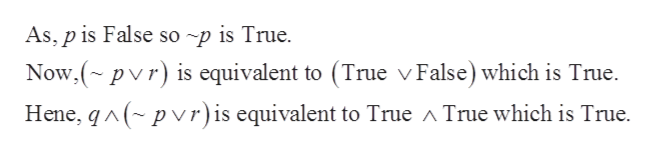# Assume that p is false, q is true, and r is false. Compute the truth value for the following expression. q ^ (~p v r)

Question

Assume that p is false, q is true, and r is false. Compute the truth value for the following expression.

q ^ (~p v r)

check_circle

Step 1

Provided that p is false, q is true, and r is false determine the truth value of the expression given below:

Step 2

The operator “Ú” logical OR results in true statement if either of the input is true.

The operator “Ù” logical AND results in true statement if and only if both the inputs are true.

The operator  “~” logical NOT negates the value of the input.

Step 3

Consider that p is false, q is true, an...help_outlineImage TranscriptioncloseAs, p is False so ~p is True Now,(~pvr) is equivalent to (True v False) which is True. p vr) is equivalent to True ^ True which is True Hene, q fullscreen

### Want to see the full answer?

See Solution

#### Want to see this answer and more?

Solutions are written by subject experts who are available 24/7. Questions are typically answered within 1 hour.*

See Solution
*Response times may vary by subject and question.
Tagged in

### Logic